# How to Calculate and Solve for Diffusivity Constant | Well Testing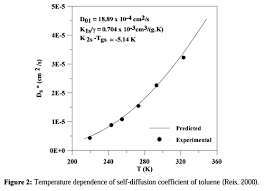The image above represents diffusivity constant.

To compute for the diffusivity constant, four essential parameters are needed and these parameters are pemerability (k), porosity (φ), viscosity (μ) and total compressibility (CT).

The formula for calculating diffusivity constant:

η = 0.000263k / φμCT

Where;

η = Diffusivity Constant
k = Permeability
φ = Porosity
μ = Viscosity
CT = Total Compressibility

Let’s solve an example;
Find the diffusivity constant where the permeability is 12, porosity is 9, viscosity is 7 and total compressibility is 11.

This implies that;

k = Permeability = 12
φ = Porosity = 9
μ = Viscosity = 7
CT = Total Compressibility = 11

η = 0.000263k /  φμCT
η = 0.000263 x 12 / 9 x 7 x 11
η = 0.003156 / 693
η = 0.000004554112554112554

Therefore, the diffusivity constant is 0.000004554112554112554.

Calculating the Permeability when the Diffusivity Constant, Porosity, Viscosity and Total Compressibility is Given.

k = n x φμCT / 0.000263

Where;

k = Permeability
η = Diffusivity Constant
φ = Porosity
μ = Viscosity
CT = Total Compressibility

Let’s solve an example;
Find the permeability where the diffusivity constant is 24, porosity is 10, viscosity is 5 and total compressibility is 13.

This implies that;

η = Diffusivity Constant = 24
φ = Porosity = 10
μ = Viscosity = 5
CT = Total Compressibility = 13

k = n x φμCT / 0.000263
k = 24 x 10 x 5 x 13 / 0.000263
k = 15600 / 0.000263
k = 59315589.3536

Therefore, the permeability is 59315589.3536.

Calculating the Porosity when the Diffusivity Constant, Permeability, Viscosity and Total Compressibility is Given.

φ = 0.000263k / nμCT

Where;

φ = Porosity
k = Permeability
η = Diffusivity Constant
μ = Viscosity
CT = Total Compressibility

Let’s solve an example;
Find the porosity where the diffusivity constant is 28, permeability is 8, viscosity is 12 and total compressibility is 18.

This implies that;

k = Permeability = 8
η = Diffusivity Constant = 28
μ = Viscosity = 12
CT = Total Compressibility = 18

φ = 0.000263k / nμCT
φ = 0.000263 x 8 / 28 x 12 x 18
φ = 0.002104 / 6048
φ = 3.478e-7

Therefore, the porosity is 3.47e-7.

Calculating the Viscosity when the Diffusivity Constant, Permeability, Porosity and Total Compressibility is Given.

μ = 0.000263k / nφCT

Where;

μ = Viscosity
k = Permeability
η = Diffusivity Constant
φ = Porosity
CT = Total Compressibility

Let’s solve an example;
Find the viscosity where the diffusivity constant is 30, permeability is 15, porosity is 6 and total compressibility is 21.

This implies that;

k = Permeability = 15
η = Diffusivity Constant = 30
φ = Porosity = 6
CT = Total Compressibility = 21

μ = 0.000263k / nφCT
μ = 0.000263 x 15 / 30 x 6 x 21
μ = 0.003945 / 3780
μ = 1.04e-6

Therefore, the viscosity is 1.04e-6.

Calculating the Total Compressibility when the Diffusivity Constant, Permeability, Porosity and Viscosity is Given.

CT = 0.000263k / nφμ

Where;

CT = Total Compressibility
k = Permeability
η = Diffusivity Constant
φ = Porosity
μ = Viscosity

Let’s solve an example;
Find the total compressibility where the diffusivity constant is 19, permeability is 14, porosity is 11 and  viscosity is 4.

This implies that;

k = Permeability = 14
η = Diffusivity Constant = 19
φ = Porosity = 11
μ = Viscosity = 4

CT = 0.000263k / nφμ
CT = 0.000263 x 14 / 19 x 11 x 4
CT = 0.0003682 / 836
CT = 4.04e-7

Therefore, the total compressibility is 4.04e-7.

Nickzom Calculator – The Calculator Encyclopedia is capable of calculating the diffusivity constant.

To get the answer and workings of the diffusivity constant using the Nickzom Calculator – The Calculator Encyclopedia. First, you need to obtain the app.

You can get this app via any of these means:

To get access to the professional version via web, you need to register and subscribe for NGN 1,500 per annum to have utter access to all functionalities.
You can also try the demo version via https://www.nickzom.org/calculator

Apple (Paid) – https://itunes.apple.com/us/app/nickzom-calculator/id1331162702?mt=8
Once, you have obtained the calculator encyclopedia app, proceed to the Calculator Map, then click on Petroleum under EngineeringNow, Click on Well Testing under Petroleum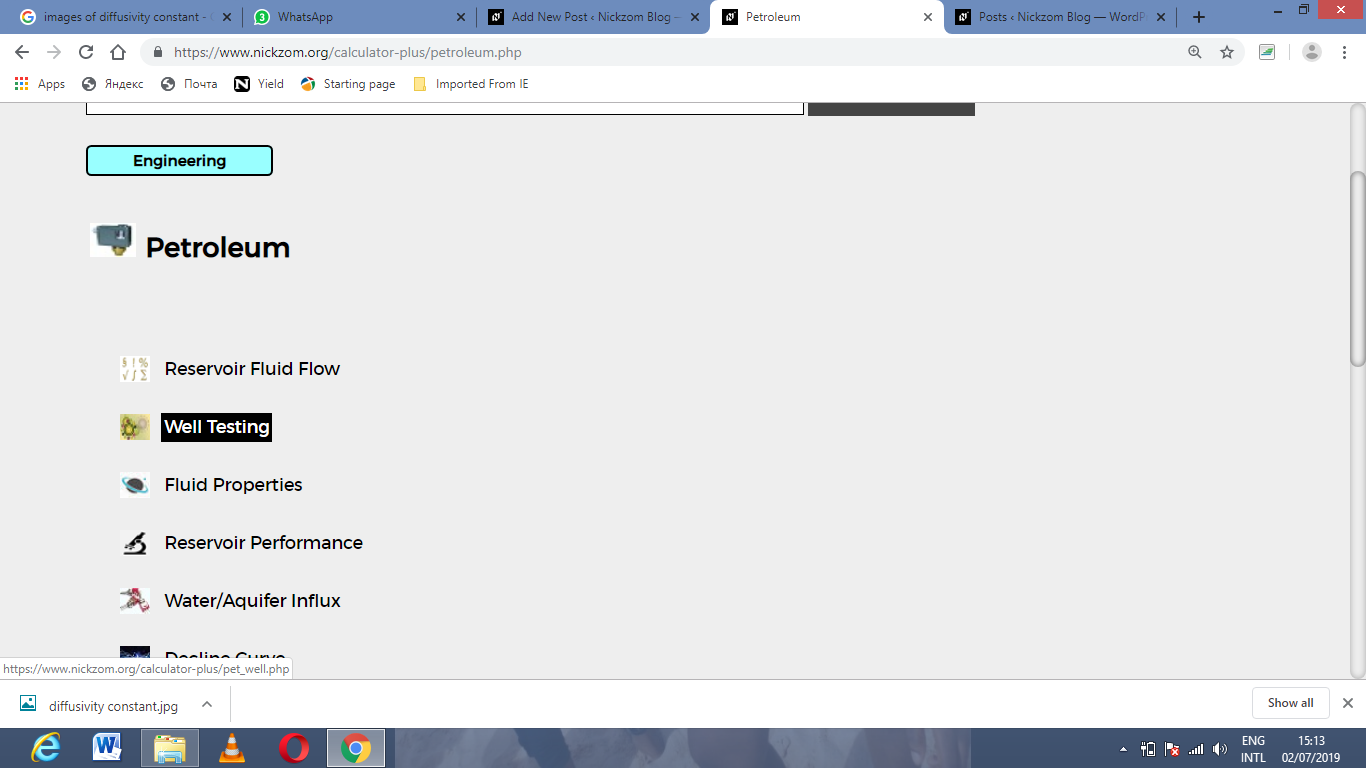Now, Click on Diffusivity Constant under Well Testing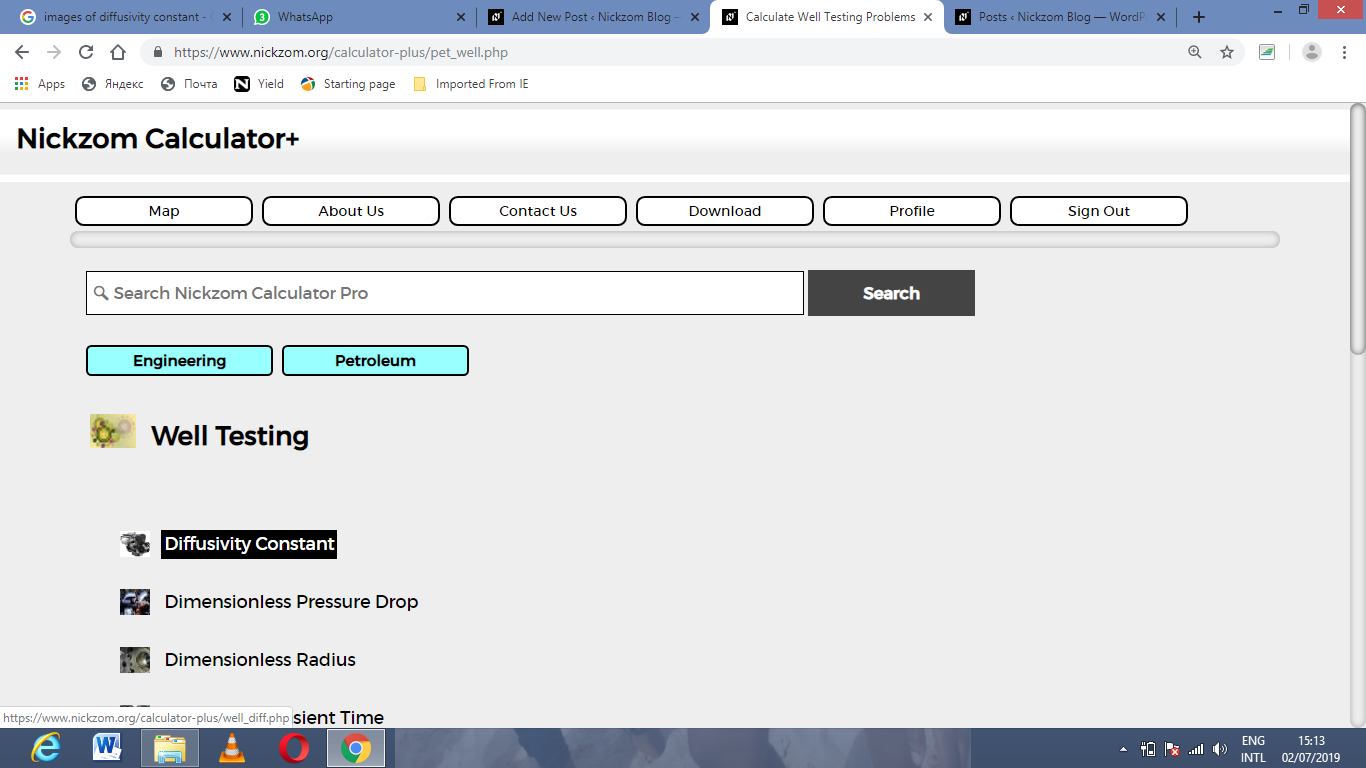The screenshot below displays the page or activity to enter your value, to get the answer for the diffusivity constant according to the respective parameter which are the pemerability (k), porosity (φ), viscosity (μ) and total compressibility (CT).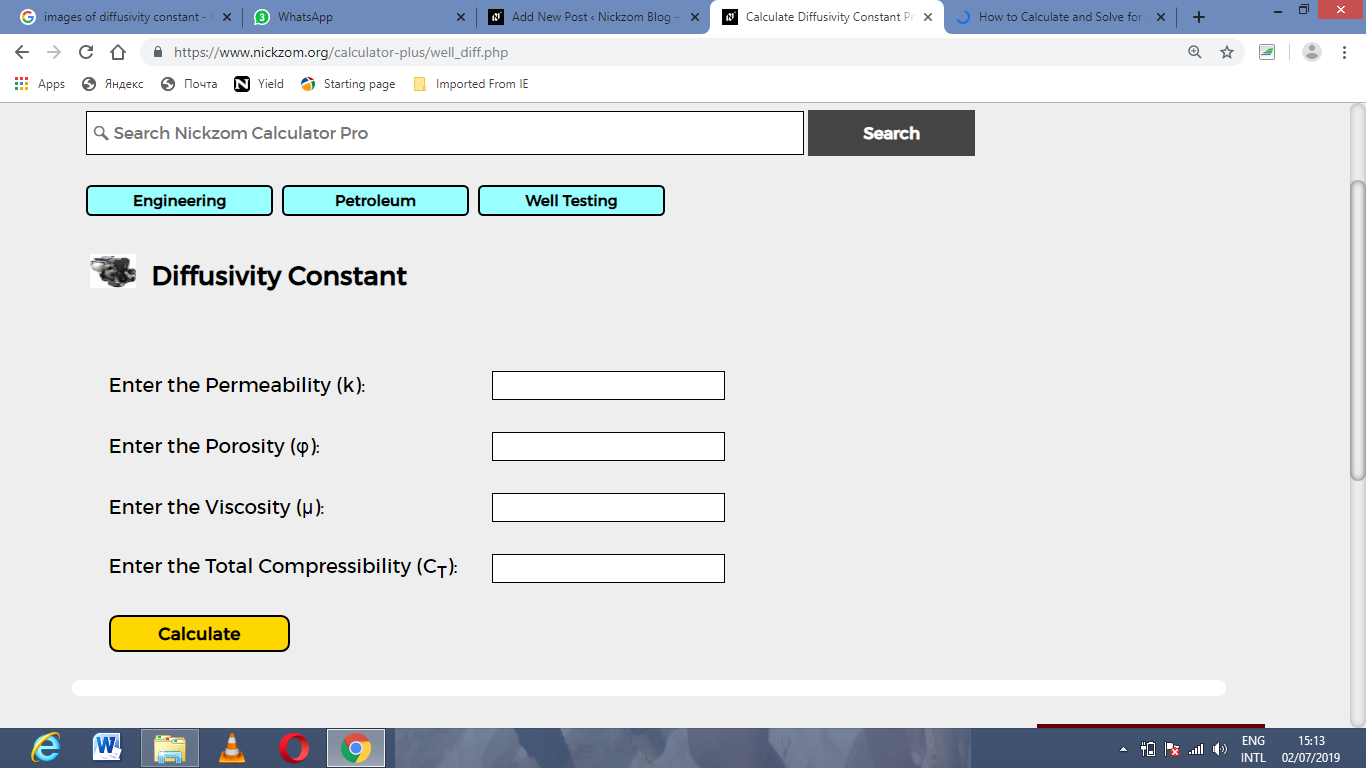Now, enter the value appropriately and accordingly for the parameter as required by the pemerability (k) is 12, porosity (φ) is 9, viscosity (μ) is 7 and total compressibility (CTis 11.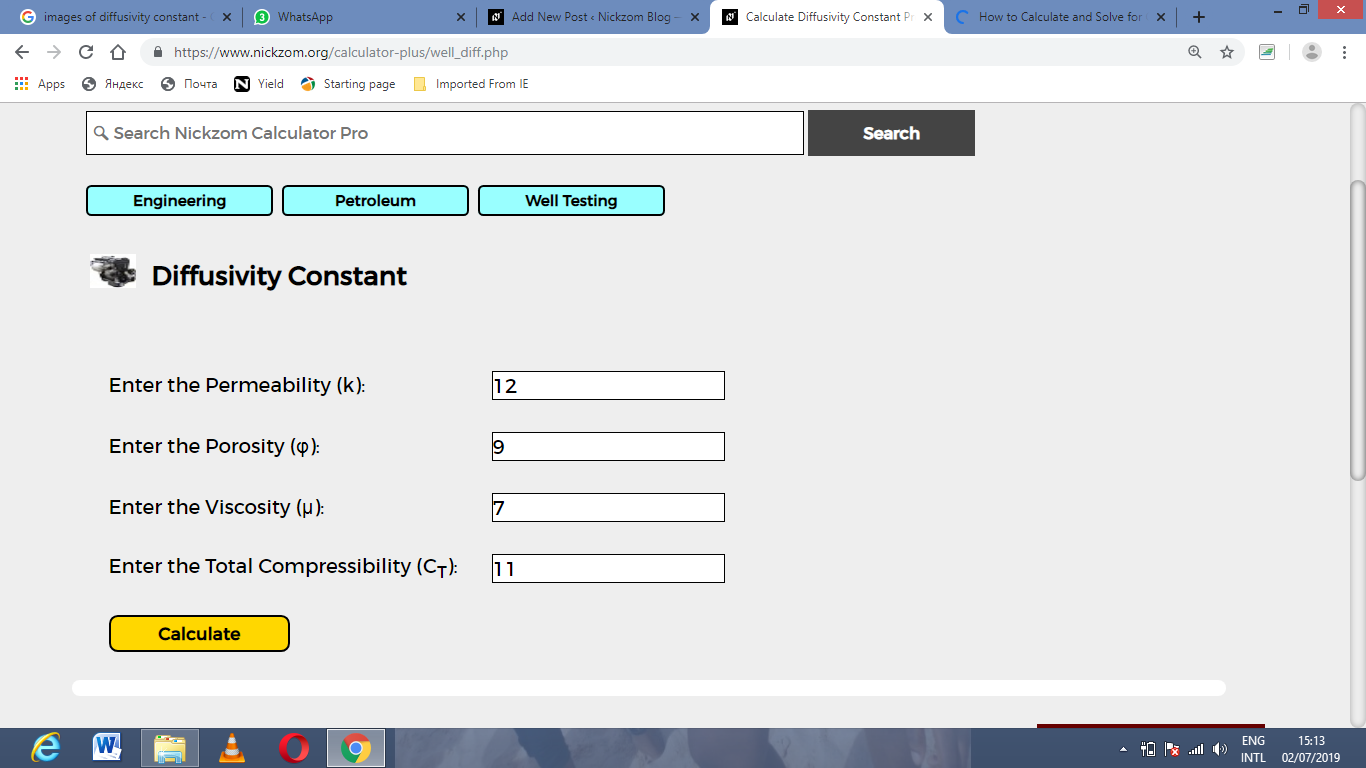Finally, Click on Calculate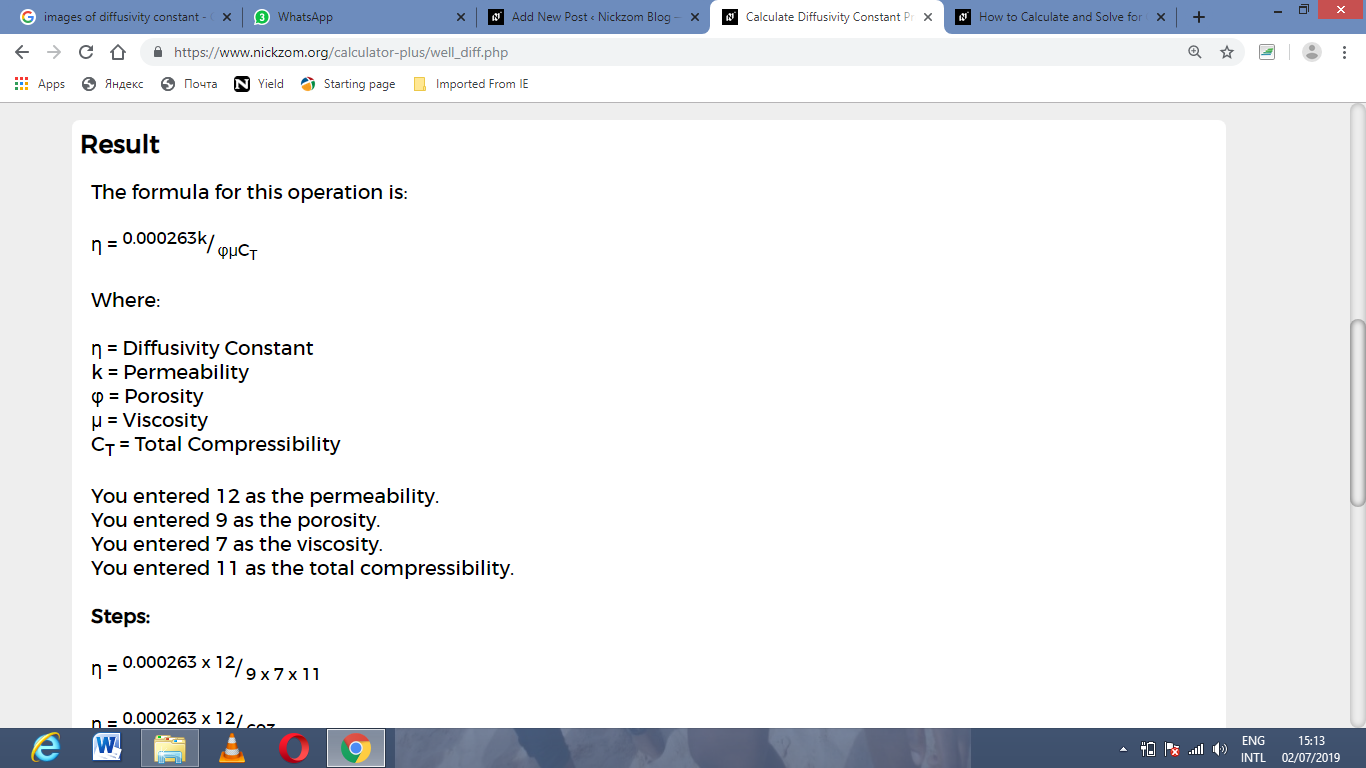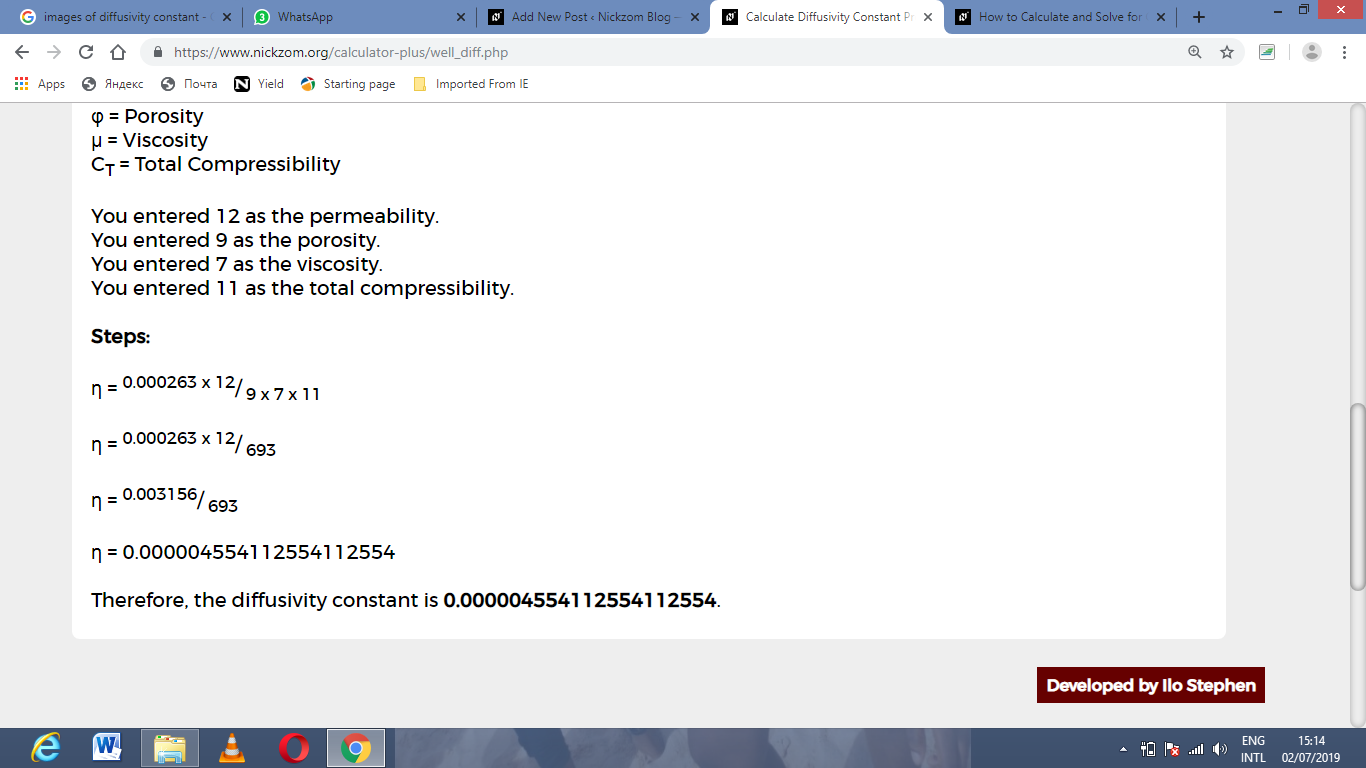As you can see from the screenshot above, Nickzom Calculator– The Calculator Encyclopedia solves for the diffusivity constant and presents the formula, workings and steps too.